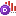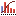AnyLogic

# Histogram data

Histogram Data object does the following:

• Performs standard statistical analysis on the data values being added (calculates mean, minimum, maximum, deviation, variance, and mean confidence interval).
• Builds the PDF (probability distribution, or density function).
• May calculate (depends on the user choice) CDF (cumulative distribution function) on the fixed set of intervals defined by the user and the lower and higher confidence measures (or values at risk with a given percent) with tolerance equal to the interval width.

The collected statistics can be visualized with the Histogram object.

You can set the Histogram data element to write data into the model execution log — histograms_log. To do this, select the element's option Log to database, and enable the model to write to the log as described in Model execution logs.

1. Drag theHistogram Data element from theAnalysis palette into the graphical editor.
2. Go to the Properties view.
3. Enter the expression you want to collect statistics over in the Value edit box.
4. If you want CDF to be calculated, select the Calculate CDF check box.
5. If you want percentiles to be calculated, select the Calculate percentiles check box and specify low and high confidence measures in the Low and High edit boxes correspondingly.
6. Define histogram intervals. Using the radio buttons in the section Values range, choose whether you want to define intervals for the data by yourself, or use auto-ranging.
7. If you are aware about minimum and maximum of the data values, you may define the data range limits and the number of intervals statically. In this case choose Fixed option and explicitly specify Minimum and Maximum values and the number of intervals in the Number of intervals edit box in the properties above.
8. Otherwise, choose auto-ranging option. Auto-ranging does not require the user to pre-define the range of data. Instead, the object will automatically adjust the intervals to the actual data being added. The user only needs to specify the number of intervals and the initial interval width. In this case choose Automatically detected and specify the Initial interval size.
9. Finally, choose, how you want this data element to be updated.

## Properties

General

Name — The name of the histogram data. The name is used to identify and access this analysis data object.

Ignore — If selected, this analysis data object is excluded from the model.

Visible — If selected, the analysis data object is visible on the presentation at runtime.

Show name — If selected, the name of this analysis data object is displayed on a presentation diagram.

Value — The expression dynamically evaluating the data object value.

Number of intervals — The number of intervals of the histogram.

Calculate CDF — If selected, CDF (cumulative distribution function) is calculated.

Calculate percentiles — If selected, percentiles are calculated. In this case, specify Low and High confidence measures.

Log to database — If selected, data collected by this histogram data element will be added into the model execution log — histograms_log (if logging is turned on in the model’s Database properties).

Values range

Values range — Specify here, whether values range is Fixed with statically defined Minimum and Maximum, or Automatically detected with the Initial interval size.

Data update

Do not update data automatically — If selected, data samples are not updated automatically. In this case you should add new samples by yourself as described in Updating analysis data objects.

Update data automatically — If selected, new data samples are added automatically with the specified Recurrence time. Also, you can define here whether you want to Use model time or Use calendar dates. Depending on this choice, you can specify when updating begins with either First update time or Update date properties.

## API for working with collected data

You can work with collected data using the following functions. Histogram data element is represented in AnyLogic with instance of the following classes — HistogramSimpleData and HistogramSmartData.

HistogramSimpleData
Data of a histogram with fixed minimum, maximum, and number of intervals. The outlaying samples are registered in special “too low” and “too high” intervals. This class provides the following functions:
Function Description
double getPDFOutsideHigh() Returns the percent of samples (0..1) higher than the specified maximum.
double getPDFOutsideLow() Returns the percent of samples (0..1) lower than the specified minimum.
void setMinMax(double min, double max) Fully resets the histogram data and sets the new range covered by intervals.
HistogramSmartData
Data of a histogram with a fixed number of intervals but auto-adjustable data range. The data range covered by the intervals always includes the full range of data samples added. This class provides the following functions:
Function Description
double getIntervalWidth() Returns the current interval width, i.e. the data range corresponding to one interval.
double getLowerBound() Returns the lower bound of the range covered by intervals.

Both classes are inherited from the base class HistogramData that provides the most frequently used functions common for both types of histograms.

Common functions
Function Description
void add(double val) Adds a sample data item to the histogram data.

val — the sample value.
int count() Returns the number of samples added to the histogram data.
void reset() Fully resets the histogram data: discards all PDF/CDF data and statistics.
double max() Returns the maximum sample value, or -infinity if no samples have been added.
double mean() Returns the mean of the histogram.
double meanConfidence() Returns the half-width mean confidence interval of the histogram data. Mean confidence interval is calculated in assumption that confidence level is equal to 95%.
double min() Returns the minimum sample value, or infinity if no samples have been added.
double deviation() Returns the standard deviation of the histogram data.
int getNumberOfIntervals() Returns the number of intervals in the histogram data.
StatisticsDiscrete getStatistics() Returns the statistics object embedded into the histogram data.
double getXMax() Returns the upper bound of the range covered by intervals.
double getXMin() Returns the lower bound of the range covered by intervals.
PDF
Function Description
double getPDF(int index) Returns the PDF (probability distribution function) at the given interval.
double getMaxPDF() Returns the maximum PDF value across all intervals, i.e. the maximum number of hits per interval divided by the total number of samples.
CDF
Function Description
double getCDF(int index) Returns the CDF (cumulative distribution function) at the given interval.
void setCDFEnabled(boolean yes) Enables or disables the CDF calculation.
boolean isCDFEnabled() Checks if the CDF calculation is enabled. Returns true if CDF calculation is enabled, false otherwise.
Percentiles
Function Description
boolean arePercentilesEnabled() Checks if percentile calculation is enabled. Returns true if percentile calculation is enabled, false otherwise.
void setPercentilesEnabled(boolean yes) Enables or disables calculation of percentiles (the data values corresponding to a certain low and high percent bounds).
void setPercents(double low, double high) Sets the percent bounds for percentile calculation.
double getPercentHigh() Returns the high percent value used for percentile calculation (1 is 100%).
double getPercentLow() Returns the low percent value used for percentile calculation (1 is 100%).

## Troubleshooting

If you run your model and cannot see the CDF line on your histogram (while the Show CDF checkbox is selected) — please open the properties of the Histogram Data object displayed by your histogram and select the Calculate CDF checkbox there.